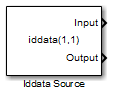Documentation

### This is machine translation

Mouseover text to see original. Click the button below to return to the English version of the page.

Note: This page has been translated by MathWorks. Click here to see
To view all translated materials including this page, select Country from the country navigator on the bottom of this page.

# Iddata Source

Import time-domain data stored in `iddata` object in MATLAB workspace

• Library:
• System Identification Toolbox## Description

The Iddata Source block imports the input-output time-domain data stored in an `iddata` object in the MATLAB® workspace. You can use this block to import data for simulating a model in Simulink®.

## Ports

### Output

expand all

Input data stored in `iddata` object, returned as a scalar for single-input data and a vector of length Nu for multichannel data with Nu inputs. If `z` is the `iddata` object, the output at this port at simulation time t is `z.InputData` at time t. If t is greater than `z.SamplingInstants(end)`, the maximum time in `z`, the input data is returned as `0` for each input channel.

Data Types: `double`

Output data stored in `iddata` object, returned as a scalar for single-output data and as a vector of length Ny for multichannel data with Ny outputs. If `z` is the `iddata` object, the output at this port at simulation time t is `z.OutputData` at time t. If t is greater than `z.SamplingInstants(end)`, the maximum time in `z`, the output data is returned as `0` for each output channel.

Data Types: `double`

## Parameters

expand all

Time-domain data that is to be imported, specified as an `iddata` object that you have created in the MATLAB workspace. The `iddata` object must contain only one experiment. For a multiple-experiment `iddata` object `z`, to specify an `iddata` object for experiment number `kexp`, specify IDDATA object as `getexp(z,kexp)`.

## See Also

### Topics

#### Introduced in R2008a

##### SupportGet trial now